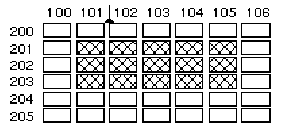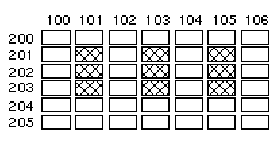Oracle® Developer Studio 12.5: Debugging a Program with dbx
Updated: June 2016

## Evaluating Arrays

You evaluate arrays the same way you evaluate other types of variables.

The following example is a sample Fortran array:

`integer*4 arr(1:6, 4:7)`

To evaluate the array, use the print command. For example:

`(dbx) print arr(2,4)`

The dbx print command enables you to evaluate part of a large array. Array evaluation includes:

• Array slicing – Prints any rectangular, n-dimensional box of a multidimensional array.

• Array striding – Prints certain elements only, in a fixed pattern, within the specified slice, which might be an entire array.

You can slice an array, with or without striding. (The default stride value is 1, which means print each element.)

### Array Slicing

Array slicing is supported in the print, display, and watch commands for C, C++, and Fortran.

#### Array Slicing Syntax for C and C++

For each dimension of an array, the full syntax of the print command to slice the array is as follows:

`print array-expression [first-expression .. last-expression : stride-expression]`

where:

array-expression

Expression that should evaluate to an array or pointer type.

first-expression

First element to be printed. Defaults to 0.

last-expression

Last element to be printed. Defaults to upper bound.

stride-expression

Length of the stride (the number of elements skipped is stride-expression-1). Defaults to 1.

The first expression, last expression, and stride expression are optional expressions that should evaluate to integers.

For example:

```(dbx) print arr[2..4]
arr[2..4] =
 = 2
 = 3
 = 4
(dbx) print arr[..2]
arr[0..2] =
 = 0
 = 1
 = 2

(dbx) print arr[2..6:2]
arr[2..6:2] =
 = 2
 = 4
 = 6```

#### Array Slicing Syntax for Fortran

For each dimension of an array, the full syntax of the print command to slice the array is as follows:

`print array-expression (first-expression : last-expression : stride-expression)`

where:

array-expression

Expression that should evaluate to an array type.

first-expression

First element in a range, also first element to be printed. Defaults to lower bound.

last-expression

Last element in a range, but might not be the last element to be printed if stride is not equal to 1. Defaults to upper bound.

stride-expression

Length of the stride. Defaults to 1.

The first expression, last expression, and stride expression are optional expressions that should evaluate to integers. For an n-dimensional slice, separate the definition of each slice with a comma.

For example:

```(dbx) print arr(2:6)
arr(2:6) =
(2) 2
(3) 3
(4) 4
(5) 5
(6) 6

(dbx) print arr(2:6:2)
arr(2:6:2) =
(2) 2
(4) 4
(6) 6```

To specify rows and columns:

```demo% f95 -g -silent ShoSli.f
demo% dbx a.out
Reading symbolic information for a.out
(dbx) list 1,12
1         INTEGER*4 a(3,4), col, row
2         DO row = 1,3
3             DO col = 1,4
4               a(row,col) = (row*10) + col
5             END DO
6         END DO
7         DO row = 1, 3
8                WRITE(*,’(4I3)’) (a(row,col),col=1,4)
9        END DO
10        END
(dbx) stop at 7
(1) stop at "ShoSli.f":7
(dbx) run
Running: a.out
stopped in MAIN at line 7 in file "ShoSli.f"
7          DO row = 1, 3```

To print row 3:

```(dbx) print a(3:3,1:4)
’ShoSli’MAIN’a(3:3, 1:4) =
(3,1)   31
(3,2)   32
(3,3)   33
(3,4)   34
(dbx)```

To print column 4:

```(dbx) print a(1:3,4:4)
’ShoSli’MAIN’a(1:3, 1:4) =
(1,4)   14
(2,4)   24
(3,4)   34
(dbx)```

### Using Slices

The following example is a two-dimensional, rectangular slice of a C++ array, with the default stride of 1 omitted.

`print arr[201..203][101..105]`

This command prints a block of elements in a large array. Note that the command omits stride-expression, using the default stride value of 1.As illustrated, the first two expressions (201:203) specify a slice in the first dimension of this two-dimensional array (the three-row column). The slice starts with row 201 and ends with 203. The second set of expressions, separated by a comma from the first, defines the slice for the second dimension. The slice begins with column 101 and ends with column 105.

### Using Strides

When you instruct print to stride across a slice of an array, dbx evaluates certain elements in the slice only, skipping over a fixed number of elements between each one it evaluates.

The third expression in the array slicing syntax, stride-expression, specifies the length of the stride. The value of stride-expression specifies the elements to print. The default stride value is 1, meaning: evaluate all of the elements in the specified slices.

The following example is the same array used in the previous example of a slice. This time, the print command includes a stride of 2 for the slice in the second dimension.

`print arr(201:203, 101:105:2)`

As shown in the diagram, a stride of 2 prints every second element, skipping every other element.For any expression you omit, print takes a default value equal to the declared size of the array. The following examples show how to use the shorthand syntax.

For a one-dimensional array, use the following commands:

print arr

Prints the entire array with default boundaries.

print arr(:)

Prints the entire array with default boundaries and default stride of 1.

print arr(::stride-expression)

Prints the entire array with a stride of stride-expression.

For a two-dimensional array, the following command prints the entire array.

`print arr`

The following command prints every third element in the second dimension of a two-dimensional array:

`print arr (:,::3)`